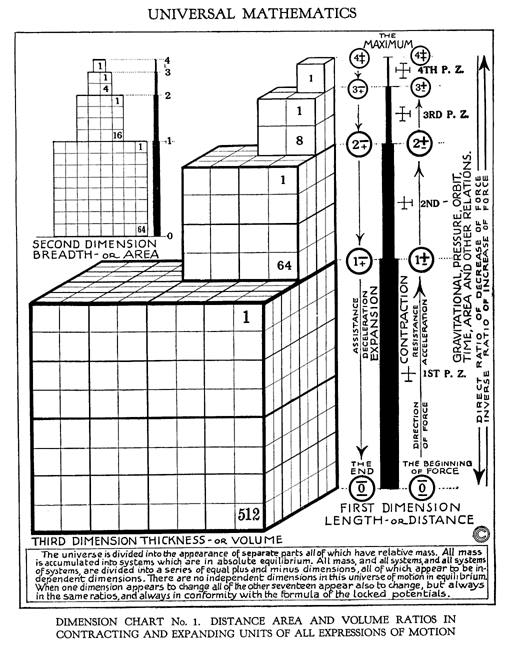# volume

noun: the loudness of a sound from a television, radio, etc.
noun: an amount of something
noun: the amount of space something fills, or the amount of space in a container
noun: the magnitude of sound (usually in a specified direction) ("The kids played their music at full volume")
noun: the amount of 3-dimensional space occupied by an object ("The gas expanded to twice its original volume")
noun: a relative amount ("Mix one volume of the solution with ten volumes of water")
noun: the property of something that is great in magnitude

"...Volume is perceptible Space. Everything in the Universe has Volume, and there exists no perceptible Space other than that of Volume." [R. A. Schwaller de Lubicz, The Temple in Man]Figure 12.09 - Dimensions and Their RelationshipsFigure 12.09 - Dimensions and Their Relationships

In graphic Figure 12.09 - Dimensions and Relationships it is clear:

Relative Volume

Accumulating Dispersing

4+ = 1/8 of 3+ or 3+ = 8 X 4+ or 81

3+ = 1/8 of 2+ or 2+ = 8 X 3+ or 82

2+ = 1/8 of 1+ or 1+ = 8 X 2+ or 83

Numeric Progressions (units)

1st Dimension = Linear = 1, 2, 4, 8.. (Doubling, nX2)

2nd Dimension = Area = 1, 4, 8, 64.. (Squaring, n2)

3rd Dimension = Volume = 1, 8, 64, 512.. (Cubing, n3)

Volumes

Cube Volume = 1 = 13 = 1 on a side

Cube Volume = 2 = cube root of 2 = 1.259922 on side

Cube Volume = 4 = cube root of 4 = 1.587403 on side

Cube Volume = 8 = cube root of 8 = 2 on side

therefore

Wavelengths and Frequencies - Octave Relations of Russell's Indig Number System

 Indig Vol. Units Vol. Calc Wavelength Example Octave Note 4 1 13 1 1 cps 4 G as 4th octave 3 8 23 2 1/2 cps 3 F as 3rd octave 2 64 43 4 1/4 cps 2 E as 2nd octave 1 512 83 8 1/8 cps 1 D as 1st octave 0 C## non-octave

Table 12.02.01 - Wavelengths and Frequencies

Showing linear versus geometric progressions as also other types of progressions (counting methods or scales).